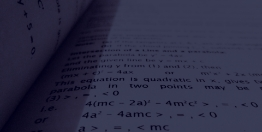# MCAT Physics Equations Sheet

## Gold Standard's MCAT Physics Equations Sheet ('cheat sheet' notes, formulas)

This MCAT Physics Equations Sheet provides helpful physics equations for exam preparation. Physics equations on motion, force, work, energy, momentum, electricity, waves and more are presented below. Please keep in mind that understanding the meaning of equations and their appropriate use will always be more important than memorization. Sometimes, formulas will be provided during the exam but, as you will see, you are expected to already know quite a few. Doing and reviewing practice questions and practice tests will improve your understanding of what you need to know.

If you don't see the Greek symbols - alpha: α;   mu: μ;  delta: Δ; - then the equations on this page will not make sense; thus, adjust fonts on your browser to Unicode.

Download the Gold Standard MCAT Physics Equations Sheet PDF for FREE (no registration required), or scroll down and click on an image to view the equations.

## Watch a Physics Video from Our MCAT Videos Library

To access more physics videos:

#### MCAT PHYSICS EQUATIONS TO MEMORIZEMCAT Physics equations from translational motion to refraction

#### MCAT PHYSICS EQUATIONS TO MEMORIZE AS PAIRSPhysics equations (formulas) to memorize as pairs

#### MCAT PHYSICS EQUATIONS TO KNOW HOW TO USEPhysics equations from Bernouilli's Equation to Doppler Effect

#### ATOMIC NUCLEUS AND ELECTRONIC STRUCTUREPhysics equations on atomic nuclear and electronic structure.

#### THE BASICS OF TRIGONOMETRY ON THE EXAMLearn the sin, cos and tan basics in Trigonometry

#### MCAT PHYSICS UNITS TO MEMORIZEMCAT Physics units like work, energy, SI units and more.

#### TAKE A FREE FULL-LENGTH PRACTICE TEST#### GOLD STANDARD PREPHOME STUDY AND ONLINE PREPPRACTICE TESTSQUESTION BANKS REVIEWCourses with clear teaching and more than 10,000 Q&As.

## Do you have to memorize all equations for MCAT?

No, you do not need to memorize all equations for the MCAT. For MCAT Physics, there are many equations that you should memorize in order to maximize your score. Occasionally, the AAMC will provide physics formulas during the exam itself, but never basic formulas.

## How do you remember MCAT physics formulas?

The best way to remember MCAT Physics formulas is by: 1) understanding the idea(s) behind the equation; 2) application: doing and reviewing practice questions and practice tests that require classic MCAT physics formulas, which of course, will improve your understanding of what you need to know; 3) in rare instances, using mnemonics or other memory devices (e.g. heat energy Q = mcΔT = MCAT !).

## How hard is MCAT Physics?

Yes, MCAT physics is hard, but with adequate content review and consistent practice, it is quite manageable. This exam tests your knowledge on common physics concepts taught in undergraduate school, so its questions should not be any harder than the questions you faced in your college physics classes. What students tend to find most challenging about this exam’s physics content is the dimensional analysis, unit conversion, and mental math required to answer questions quickly. This is where content review and practice questions become most beneficial. The more you review the formulas and units, the less hard this section will feel.

## Is the MCAT Physics 1 or 2?

Physics topics on the MCAT usually cover content from the first semester of college physics, or Physics 1, and the second semester of college physics, or Physics 2. For curriculums in which Physics 2 covers more theoretical applications, this exam will focus primarily on topics covered in Physics 1. Students who have only taken an Introduction to Physics course will most likely need to take the next-level physics course offered at their school to learn all the physics concepts tested on the exam. Regardless of the courses you have taken, make sure to review the AAMC’s exam topic outline.